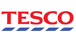Screen for any % Price Oscillator (PPO) Stocks in the Market

# % Price Oscillator (PPO)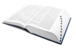What is the definition of % Price Oscillator (PPO)?

This is a momentum indicator based on the difference between two moving averages expressed as a percentage. The PPO is found by subtracting the longer moving average (26 day EMA) from the shorter moving average (12 day EMA) and then dividing the difference by the longer moving average.

It is similar to the MACD. As with its cousin, MACD, the Percentage Price Oscillator is shown with a signal line (the 9 day EMA), a histogram and a centerline. While MACD measures the absolute difference between two moving averages, PPO makes this a relative value by dividing difference by the slower moving average (26-day EMA).Stockopedia explains % Price Oscillator (PPO)...

Entry Signals are generated with crossovers of the PPO line and the signal line, the histogram and divergences. As with MACD, the PPO reflects the convergence and divergence of two moving averages. PPO is positive when the shorter moving average is above the longer moving average, reflecting upside momentum. The PPO is negative when the shorter moving average is below the longer moving average.

## Technical Indicators Ratios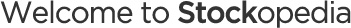Let’s get you setup so you get the most out of our serviceBrilliant - You've created a folio! Now let's add some stocks to it.
•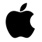Apple (AAPL)
•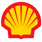Shell (RDSA)
•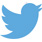•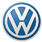•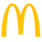•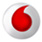•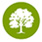•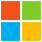•